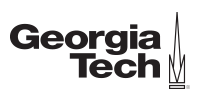Ir al contenido principal

# Learn probability concepts that can be applied in your careerCertificación Profesional en
Probability/Random Variables
GTx

## Lo que aprenderás

• Review Bootcamp lessons based on set theory and calculus.
• Understand underlying probability axioms.
• Apply elementary probability counting rules, including permutations and combinations.
• Become familiar with the concepts of independence and conditional probability.
• Learn how to update probabilities via Bayes Rule.
• Get introduced to discrete and continuous random variables.
• Learn about the properties of random variables, including the expected value, variance, and moment generating function.
• Study functions of random variables, and how they can be used in computer simulation applications.
• Recognize joint(two-dimensional) random variables and how to extract marginal(one-dimensional) and conditional information from them.
• Study the concepts of independence and correlation.
• Work with the R statistical package.

This certificate program consists of two mini-courses: (1) A Gentle Introduction to Probability; and (2) Random Variables – Great Expectations to Bell Curves.

The first course provides an introduction to basic probability concepts. Our emphasis is on applications in science and engineering, with the goal of enhancing modeling and analysis skills for a variety of real-world problems.

In order to make the course completely self-contained (and to bring back long-lost memories), we’ll start off with Bootcamp lessons to review concepts from set theory and calculus. We’ll then discuss the probability axioms that serve as the basis for all of our subsequent work – what makes probability tick?

The next venues on our tour are the concepts of independence and conditional probability, which allow us to see how the probabilities of different events are related to each other, and how new information can be used to update probabilities. The course culminates in a discussion of Bayes Rule and its various interesting consequences related to probability updates.

The second course discusses properties and applications of random variables.

We’ll begin by introducing the concepts of discrete and continuous random variables. For instance, how many customers are likely to arrive in the next hour (discrete)? What’s the probability that a lightbulb will last more than a year (continuous)?

We’ll learn about various properties of random variables such as the expected value, variance, and moment generating function. This will lead us to a discussion of functions of random variables. Such functions have many uses, including some wonderful applications in computer simulations.

If you enjoy random variables, then you’ll really love joint (two-dimensional) random variables. We’ll provide methodology to extract marginal (one-dimensional) and conditional information from these big boys. This work will enable us to study the important concepts of independence and correlation.

Along the way, we’ll start working with the R statistical package to do some of our calculations and analysis.

By the end of the course, you will have the technology to model and evaluate a variety of real-world systems in which randomness is present.

Capacitación de la mano de expertos
2 cursos de capacitación
A tu ritmo
Avanza a tu ritmo
2 meses
6 - 11 horas por semana
398 US\$
Para obtener la experiencia completa del programa

1. ## Certificación Profesional en Probability/Random Variables de GTx

2. 6–10 horas por semana durante 3 semanas

This course provides an introduction to basic probability concepts. Our emphasis is on applications in science and engineering, with the goal of enhancing modeling and analysis skills for a variety of real-world problems.

3. 6–12 horas por semana durante 4 semanas

This course discusses properties and applications of random variables. For instance, how many customers are likely to arrive in the next hour? What’s the probability that a lightbulb will last more than a year?

When you’re done with this course, you’ll have enough firepower to undertake a wide variety of modeling and analysis problems; and you’ll be well-prepared for the upcoming Statistics courses.

• This course is ideal for folks in business, industry, and science, who seek to use probability and statistics in wide-ranging application areas – everything from engineering to agriculture to psychology to sports analytics to healthcare.

## Conoce a tu instructor de The Georgia Institute of Technology (GTx)

David Goldsman
Professor of Industrial and Systems Engineering
The Georgia Institute of Technology

Expertos de GTx comprometidos con el aprendizaje en línea

## Inscríbete ahora

398 US\$
2 cursos en 2 meses

Comienza con data science

Explorar otros cursos de data science